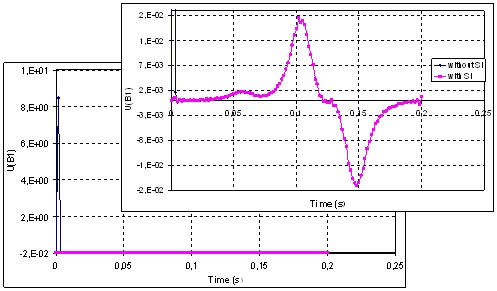# Initialization by static calculation: operation

## Static initialization

To avoid this problem of “numeric transient”, initial conditions of the « Initialization by static calculation » type can be taken into account with Flux. The goal of this option is to initialize a time computation in order to avoid transient phenomena with no physical meaning.

These calculation conditions are presented in the following blocks.

## Conditions of calculation

The initialization by static calculation is characterized by particular calculation conditions for the first time step: conditions, which permit to annul all the terms in d/dt at the step t = 0 s.

These conditions are summed up in the table below.

Element of the device Hypotheses / conditions of calculation at t = 0 s
in the finite elements domain in the electric circuit
Solid conductor Active (circuit coupling)

skin effects, proximity effects are not taken into consideration (= non magnetic conducting regions)

DC resistance (electric conduction resistance)

passive (no circuit coupling)

Induced currents are not taken into consideration (= non conducting magnetic region)

-
Coil conductor

Current in coil (=circuit current)

resistance (of coil conductor)

Element of the device in the electric circuit
Resistor

Resistance (values of the Resistor component)

Inductor

Inductance replaced by a resistor with small resistance

Capacitor

with initial voltage Uc

Capacitor replaced by a voltage source * (U = Uc)

without initial voltage

Capacitor replaced by an open circuit

Note: * Caution: for circuit with capacitor (with initial voltage) you must add a switch in the circuit (see “ Initialization by static calculation: particular cases”).

## Example 1

Magnet placed at the center of the coil

Finite elements description: Circuit description: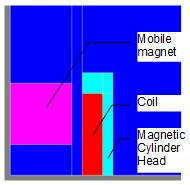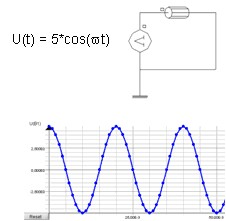Results without SI : Results with SI :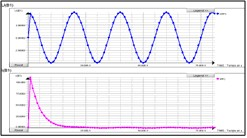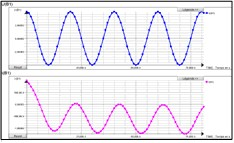## Example 2

Variable reluctance speed sensor as describe in the Generic Tutorial for Flux 3D

Finite elements description: Circuit description: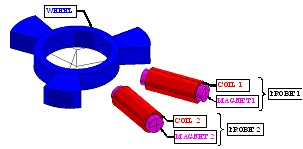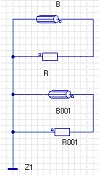Results with and without SI: